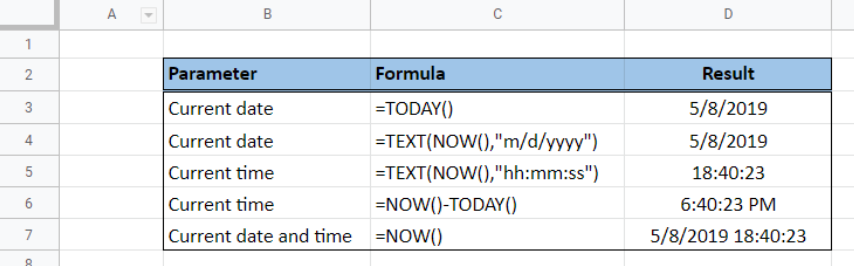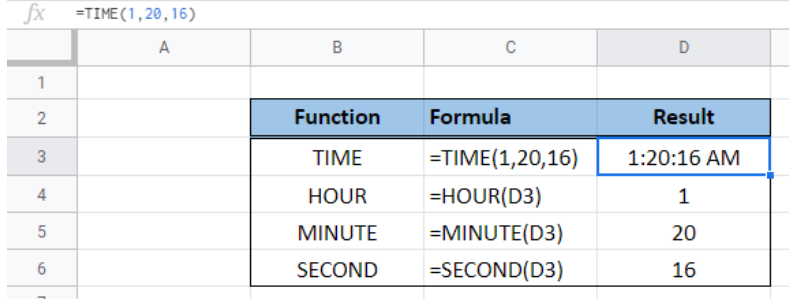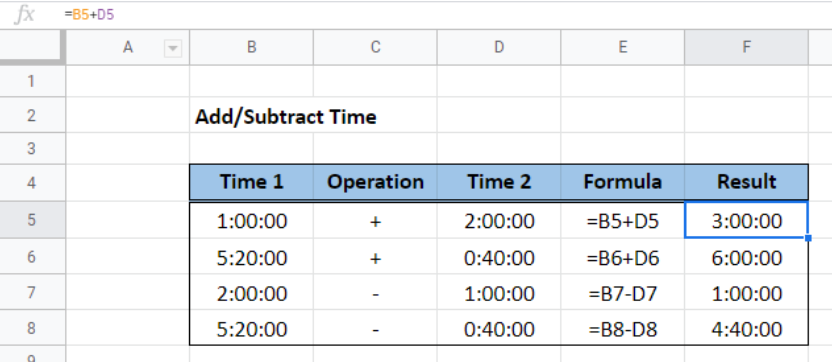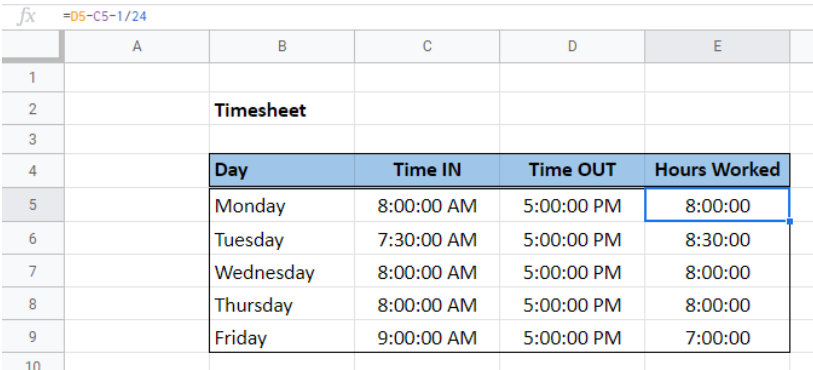Get instant live expert help with Excel or Google Sheets“My Excelchat expert helped me in less than 20 minutes, saving me what would have been 5 hours of work!”

#### Post your problem and you’ll get expert help in seconds.

Your message must be at least 40 characters
Our professional experts are available now. Your privacy is guaranteed.

# Date and time functions in Google sheets – Excelchat

Date and time functions in Google Sheets have the same usage and syntax as in Excel.  Learning the different applications of date and time formulas will be very helpful in performing related calculations that we might encounter while working with Google Sheets.

## Current date and time

The current date and time can be inserted through the functions TODAY and NOW.  TODAY function returns the current date while the NOW function returns the current date and time.  However, the NOW function can be customized to show only the date, or the time.

The below image shows the different ways to show the current date, current time or both date and time using the functions TODAY, NOW and TEXT.Figure 1.  Output: Current date and time

## Time functions

The time functions are TIME, HOUR, MINUTE and SECOND.  TIME function generates a certain time given the specific data for hour, minute and second portion of the time.  In cell D2, we are able to insert the time 1:20:16 AM by using the TIME formula

`=TIME(1,20,16)`

Where 1 is the hour, 20 is the minute and 16 is the second portion.

Conversely, we can determine the hour, minute and second portion of the time 1:20:16 AM by using the functions HOUR, MINUTE and SECOND, respectively.

The below image shows the formula and results when using time functions.Figure 2.  Output: Time Functions

In order to add or subtract time, we have to apply the basic mathematical operation addition or subtraction.  These formulas are rather straightforward and does not involve the use of any function.

To add time, we enter the first value, the plus “+” sign, then the second value.  On the other hand, in order to subtract time, we only have to change the sign from a plus sign to a minus “-”.Figure 3.  Output: Add or subtract time

## Timesheet

Timesheets are widely used to record and calculate the number of hours worked.  With spreadsheets, the task of maintaining a timesheet has become easier and more accurate.

In order to create a timesheet, we have to make a spreadsheet with a list of days, a column for Time IN, Time OUT, and hours worked.  The formula for hours worked is

`=Time_OUT - Time_IN  - unpaid_hours`

Unpaid hours could refer to lunch breaks or under time.

The below image is an example of a timesheet from Monday to Friday, with one unpaid hour every day for lunch break.  The timesheet formula is given by

`=D5-C5-1/24`

where 1/24 corresponds to one hour in a day.Figure 4.   Output: Google Sheets timesheet

## Instant Connection to an Excel Expert

Most of the time, the problem you will need to solve will be more complex than a simple application of a formula or function. If you want to save hours of research and frustration, try our live Excelchat service! Our Excel Experts are available 24/7 to answer any Excel question you may have. We guarantee a connection within 30 seconds and a customized solution within 20 minutes.